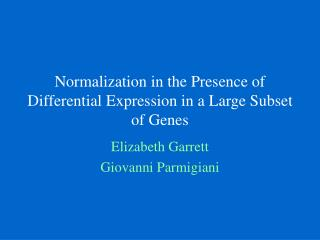DownloadDownload PresentationNormalization in the Presence of Differential Expression in a Large Subset of Genes

# Normalization in the Presence of Differential Expression in a Large Subset of Genes

Download Presentation## Normalization in the Presence of Differential Expression in a Large Subset of Genes

- - - - - - - - - - - - - - - - - - - - - - - - - - - E N D - - - - - - - - - - - - - - - - - - - - - - - - - - -
##### Presentation Transcript

1. Normalization in the Presence of Differential Expression in a Large Subset of Genes Elizabeth Garrett Giovanni Parmigiani

2. Motivation (again) • Class discovery: Find breast cancer subtypes within 81 samples of previously unclassified breast cancer tumor samples • Gene selection: Find small subset of genes which allows us to cluster tumor samples • Gene clustering: Look for genes which are differentially expressed and genes that behave similarly.

3. Raw data: log gene expression median versus log gene expression in sample i

4. Problem with raw data • “V” pattern in many of the slides • Curvature • Non-constant variance

5. “V” Patterns • Debate: • We thought…..Oops, something went wrong in the lab. We should either • correct the V’s so that we see only one line • remove the genes that are causing the V • They (i.e. “experts”) thought…..It’s REAL differential expression! • Assuming it is real, how do we normalize to straighten and stabilize variance?

6. Crude Initial Approach • Approach: • Fit a regression to each plot and identify points with large negative (positive) residuals. • Remove the genes with negative (positive) residuals (and high abundance?) and normalize using the remaining points. • Problem: Points near origin get truncated in odd way and there is no obvious way to decide how to include exclude near origin.

7. High abundance = 3 or greater

8. A “better” (and not hard to implement) approach class 0 1. Assume 2 classes of genes class 1 2. Take subset of samples where V is obvious (we picked four samples) 3. Fit a latent variable model using MCMC to predict which genes are in class 1 and which in class 0.

9. Latent Variable Model Allow different slopes and intercepts for the two classes of genes: Details:

10. Results • Goal is to estimate gene classes, cg • ’s are nuisance parameters • Based on chain, we estimate g = P(cg = 1) • at each iteration, each gene is assigned to class 0 or class 1 • by averaging class assignments over iterations, we get posterior probability of class membership • To do normalization, we restrict attention to genes with g < 0.95

11. Posterior Probabilities of Class Membership

12. Normalization • Use loess normalization where class 0 genes are the reference: rsg = residuals = ysg - loess Sample 43

13. Before and after loess normalization (R function “loess’ with weights = 1 - c_g) Before After

14. Take residuals from previous loess fit. Fit loess to squared residuals versus median Square-root of fitted value approximates standard deviation. Rescale so that overall slide variability is not lost by dividing by average slide variance. Variance Stabilization

15. Final Step Calculate normalized data: Slide median Residual from first loess gene median Variance stabilizer from second loess